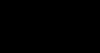# =SUM

### 1. Video

Watch the video. Don’t try to understand all the detail at this stage, just get a feel for how the formula works generally. You’ll come back to this video again later in the lesson.
You may want to expand the video to full-screen so that it’s easier to watch. Click the ‘Fullscreen’ button in the bottom right-hand corner of the player.
The video has audio so please make sure your volume is turned up.### 2. Explanation

#### Introduction

• This is the most basic formula and is extremely common. Excel’s ‘Autosum’ feature is well known, though you should be careful using this because Excel will make an assumption about which cells to include which may or may not be correct!
• =SUM simply adds up the values or cell references entered.

#### Syntax

=SUM( number1 , [number2] )

• number1: The first number to add up
• [number2]: (Optional) The second number to add up
• You can enter the numbers as cell ranges. This is the most common way of doing it in practice. Now a number of options for entering become available:-
• Individual cells can be entered, e.g. A3 or G12.
• Cell ranges can be entered, e.g. A3:A10.
• Or combinations thereof.
• Simple sums can even be entered without the ‘=SUM’ bit, e.g. =A1+B2.

#### Examples

• =SUM(10,20) will give 30
• =SUM(10,20,30) will give 60
• =SUM(A1,G3) will sum the values in cells A1 and G3
• =SUM(B5:B50) will sum all the values in the cell range B5 to B50
• =SUM(A12,A20:A25) will sum the values in cells A12, and A20 to A25
• =A3+B10-G5 will take the value in cell A3 then add on the value in cell B10 then take off the value in cell G5
• =A3*B4 will take the value in cell A3 and multiply it by the value in cell B4
• =B10/H5 will divide the value in cell B10 by the value in cell H5

#### Notes

• The format of the result is a number.
• If any cell in an included range contains a non-number then the result will be the #VALUE! error.
• If you have cells containing numbers that have been rounded using Format
• Cells, then an =SUM might appear to give the wrong answer. Actually the answer will be correct, because Excel will use the full number of decimals to calculate, even though rounded numbers appear on the screen.

### 3. Video

Watch the video through again. This time look for the details of how to create the formula and its effects on other cells, particularly the ones that are inputs to the formula.